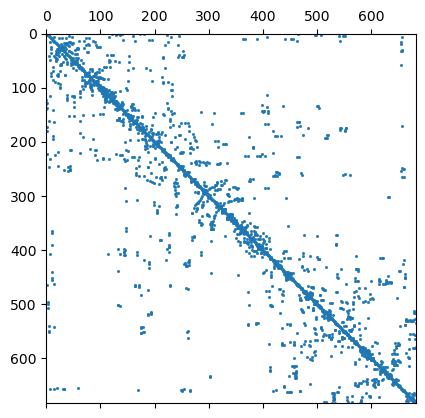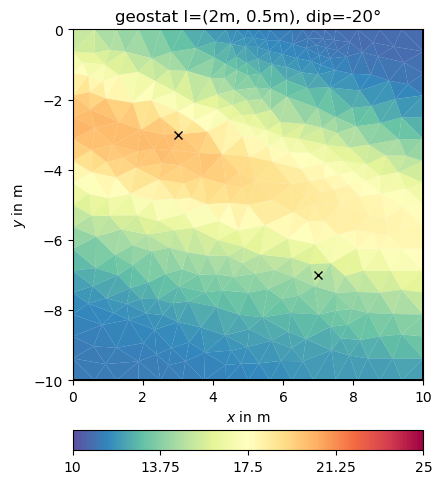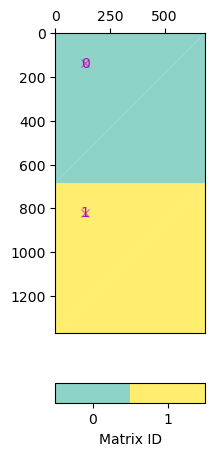# Regularization - concepts explained#

In geophysical inversion, we minimize the data objective functional as the L2 norm of the misfit between data $d$ and the forward response $f$ of the model $m$, weighted by the data error $epsilon$:

$\Phi_d = \sum\limits_i^N \left(\frac{d_i-f_i(m)}{\epsilon_i}\right)^2=\|W_d(d-f(m))\|^2$

As this minimization problem is non-unique and ill-posed, we introduce a regularization term $Phi$, weighted by a regularization parameter $lambda$:

$\Phi = \Phi_d + \lambda \Phi_m$

The regularization strength $lambda$ should be chosen so that the data are fitted within noise, i.e. $chi^2=Phi_d/N=1$.

In the term $Phi-m$ we put our expectations to the model, e.g. to be close to any prior model. In many cases we do not have much information and aim for the smoothest model that is able to fit our data. We decribe it by the operator $W_m$:

$\Phi_m=\|W_m (m-m_{ref})\|^2$

The regularization operator is defined by some constraint operator $C$ weighted by some weighting function $w$ so that $W_m=mbox{diag}(w) C$. The operator $C$ can be a discrete smoothness operator, or the identity to keep the model close to the reference model $m_{ref}$.




import numpy as np
import matplotlib.pyplot as plt
import pygimli as pg
import pygimli.meshtools as mt


Regularization drives the model where the data are too weak to constrain the model. In order to explain different kinds of regularization (also called constraints), we use a very simple mapping forward operator: The values at certain positions are picked.

from pygimli.frameworks import PriorModelling


Implementation 1. determine the indices where the cells are

ind = [mesh.findCell(po).id() for po in pos]

1. forward response: take the model at indices

response = model[ind]

1. Jacobian matrix

J = pg.SparseMapMatrix()
J.resize(len(ind), mesh.cellCount())
for i, n in enumerate(self.ind):
self.J.setVal(i, n, 1.0)


We exemplify this on behalf of a simple triangular mesh in a rectangular domain.

rect = mt.createRectangle(start=[0, -10], end=[10, 0])
mesh = mt.createMesh(rect, quality=34.5, area=0.3)
print(mesh)

Mesh: Nodes: 377 Cells: 684 Boundaries: 1060


We define two positions where we associate two arbitrary values.

pos = [[3, -3], [7, -7]]
vals = np.array([20., 15.])
fop = PriorModelling(mesh, pos)


We set up an inversion instance with the forward operator and prepare the keywords for running the inversion always the same way: - the data vector - the error vector (as relative error) - a starting model value (could also be vector)

inv = pg.Inversion(fop=fop, verbose=False)
# invkw = dict(dataVals=vals, errorVals=np.ones_like(vals)*0.03, startModel=12)
invkw = dict(dataVals=vals, relativeError=0.03, startModel=12, lam=200)
plotkw = dict(cMap="Spectral_r", cMin=10, cMax=25)


## Classical smoothness constraints#

inv.setRegularization(cType=1)  # the default
result = inv.run(**invkw)
ax, _ = pg.show(mesh, result, **plotkw)
ax.plot(pg.x(pos), pg.y(pos), "kx")

t = ax.set_title("Ctype=1")We will have a closer look at the regularization matrix $C$.

C = fop.constraints()
print(C.rows(), C.cols(), mesh)
ax, _ = pg.show(fop.constraints(), markersize=1)

row = C.row(111)
nz = np.nonzero(row)
print(nz, row[nz])992 684 Mesh: Nodes: 377 Cells: 684 Boundaries: 1060
[ 48 116] 2 [1.0, -1.0]


How does that change the regularization matrix $C$?

inv.setRegularization(cType=1, zWeight=0.2)  # the default
result = inv.run(**invkw)
ax, _ = pg.show(mesh, result, **plotkw)
ax.plot(pg.x(pos), pg.y(pos), "kx")
t = ax.set_title("Ctype=1, zWeight=0.2")

RM = fop.regionManager()
cw = RM.constraintWeights()
print(min(cw), max(cw))0.19999999999999996 1.0


Now we try some other regularization options.

inv.setRegularization(cType=0)  # damping of the model
result = inv.run(**invkw)
ax, _ = pg.show(mesh, result, **plotkw)
ax.plot(pg.x(pos), pg.y(pos), "kx")
t = ax.set_title("Ctype=1")Obviously, the damping keeps the model small ($log 1=0$) as the starting model is NOT a reference model by default. We will enable this by specifying the isReference switch.

invkw["isReference"] = True
result = inv.run(**invkw)
ax, cb = pg.show(mesh, result, **plotkw)
ax.plot(pg.x(pos), pg.y(pos), "kx")
t = ax.set_title("Ctype=0 with reference")cType=10 means a mix between 1st order smoothness (1) and damping (0)

inv.setRegularization(cType=10)  # mix of 1st order smoothing and damping
result = inv.run(**invkw)
ax, _ = pg.show(mesh, result, **plotkw)
ax.plot(pg.x(pos), pg.y(pos), "kx")
t = ax.set_title("Ctype=10")In the matrix both contributions are under each other

C = fop.constraints()
print(C.rows(), C.cols())
print(mesh)
ax, _ = pg.show(fop.constraints(), markersize=1)1676 684
Mesh: Nodes: 377 Cells: 684 Boundaries: 1060


We see that we have the first order smoothness and the identity matrix below each other. We can also use a second-order (-1 2 -1) smoothness operator by cType=2.

inv.setRegularization(cType=2)  # 2nd order smoothing
result = inv.run(**invkw)
ax, _ = pg.show(mesh, result, **plotkw)
ax.plot(pg.x(pos), pg.y(pos), "kx")
t = ax.set_title("Ctype=2")We have a closer look at the constraints matrix

C = fop.constraints()
print(C.rows(), C.cols(), mesh)
ax, _ = pg.show(C, markersize=1)684 684 Mesh: Nodes: 377 Cells: 684 Boundaries: 1060


It looks like a Laplace operator and seems to have a wider range compared to first-order smoothness.

## Geostatistical regularization#

The idea is that not only neighbors are correlated to each other but to have a wider correlation by using an operator

More details can be found in https://www.pygimli.org/_tutorials_auto/3_inversion/plot_6-geostatConstraints.html

## Application#

We can pass the correlation length directly to the inversion instance

inv.setRegularization(correlationLengths=[2, 2])
result = inv.run(**invkw)
ax, _ = pg.show(mesh, result, **plotkw)
ax.plot(pg.x(pos), pg.y(pos), "kx")
t = ax.set_title("geostat I=2m")This look structurally similar to the second-order smoothness, but can drive values outside the expected range in regions of no data coverage. We change the correlation lengths and the dip to be inclining

inv.setRegularization(correlationLengths=[2, 0.5, 2], dip=-20)
result = inv.run(**invkw)
ax, _ = pg.show(mesh, result, **plotkw)
ax.plot(pg.x(pos), pg.y(pos), "kx")
t = ax.set_title("geostat I=(2m, 0.5m), dip=-20°")We now add many more points.

np.random.seed(42) # reproducabilty is our friend
N = 30
x = np.random.rand(N) * 10
y = -np.random.rand(N) * 10
v = np.random.rand(N) * 10 + 10


and repeat the above computations

pos = [pg.Pos(xi, yi) for xi, yi in zip(x, y)]
fop = PriorModelling(mesh, pos)
inv = pg.Inversion(fop=fop, verbose=True)
inv.setRegularization(correlationLengths=[4, 4])
result = inv.run(v, relativeError=0.03, startModel=10, lam=10)
ax, _ = pg.show(mesh, result, **plotkw)
out = ax.plot(x, y, "kx")
t = ax.set_title("geostat I=4m")fop: <pygimli.frameworks.modelling.PriorModelling object at 0x2d6e95800>
Data transformation: <pygimli.core._pygimli_.RTrans object at 0x28fe4c200>
Model transformation (cumulative):
0 <pygimli.core._pygimli_.RTransLogLU object at 0x28fe4c200>
min/max (data): 10.06/19.87
min/max (error): 3%/3%
min/max (start model): 10/10
--------------------------------------------------------------------------------
inv.iter 0 ... chi² =  121.32
--------------------------------------------------------------------------------
inv.iter 1 ... chi² =    4.70 (dPhi = 95.29%) lam: 10.0
--------------------------------------------------------------------------------
inv.iter 2 ... chi² =    3.00 (dPhi = 28.65%) lam: 10.0
--------------------------------------------------------------------------------
inv.iter 3 ... chi² =    3.00 (dPhi = 0.02%) lam: 10.0
--------------------------------------------------------------------------------
inv.iter 4 ... chi² =    3.00 (dPhi = 0.00%) lam: 10.0
################################################################################
#                 Abort criterion reached: dPhi = 0.0 (< 2.0%)                 #
################################################################################


Comparing the data with the model response is always a good idea.

out = plt.plot(v, inv.response, "*")## Individual regularization operators#

Say you want to combine geostatistic operators with a damping, you can create a block matrix pasting the matric vertically.

C = pg.matrix.BlockMatrix()
G = pg.matrix.GeostatisticConstraintsMatrix(mesh=mesh, I=[2, 0.5], dip=-20)
I1 = pg.matrix.IdentityMatrix(mesh.cellCount(), val=0.1)
C.addMatrix(I1, mesh.cellCount(), 0)  # shifted down by number of cells
ax, _ = pg.show(C)Note that in pg.matrix you find a lot of matrices and matrix generators.

We set this matrix directly and do the inversion.

fop.setConstraints(C)
result = inv.run(v, relativeError=0.03, startModel=17, isReference=1, lam=10)
ax, _ = pg.show(mesh, result, **plotkw)
out = ax.plot(x, y, "kx")
t = ax.set_title("geostat + reference")fop: <pygimli.frameworks.modelling.PriorModelling object at 0x2d6e95800>
Data transformation: <pygimli.core._pygimli_.RTrans object at 0x2b3df73e0>
Model transformation (cumulative):
0 <pygimli.core._pygimli_.RTransLogLU object at 0x2b3df7140>
min/max (data): 10.06/19.87
min/max (error): 3%/3%
min/max (start model): 17/17
--------------------------------------------------------------------------------
inv.iter 0 ... chi² =  108.82
--------------------------------------------------------------------------------
inv.iter 1 ... chi² =    5.32 (dPhi = 94.94%) lam: 10.0
--------------------------------------------------------------------------------
inv.iter 2 ... chi² =    2.97 (dPhi = 41.41%) lam: 10.0
--------------------------------------------------------------------------------
inv.iter 3 ... chi² =    2.96 (dPhi = 0.11%) lam: 10.0
--------------------------------------------------------------------------------
inv.iter 4 ... chi² =    2.96 (dPhi = 0.00%) lam: 10.0
################################################################################
#                 Abort criterion reached: dPhi = 0.0 (< 2.0%)                 #
################################################################################


If you are using a method manager, you access the inversion instance by mgr.inv and the forward operator by mgr.fop.

Note

Take-away messages

• regularization drives the model where data are weak

• think and play with your assumptions to the model

• there are several predefined options

• geostatistical regularization can be superior, because: - it is mesh-independent - it better fills the data gaps (e.g. 3D inversion of 2D profiles)

Gallery generated by Sphinx-Gallery